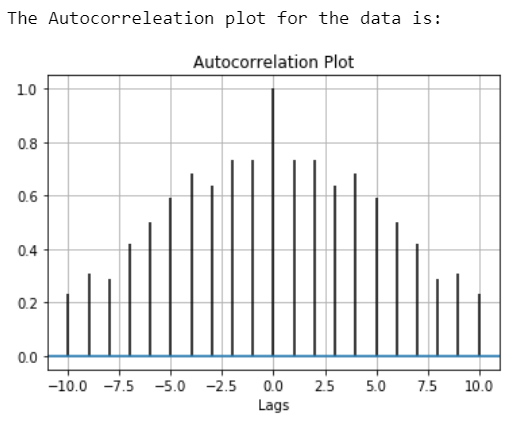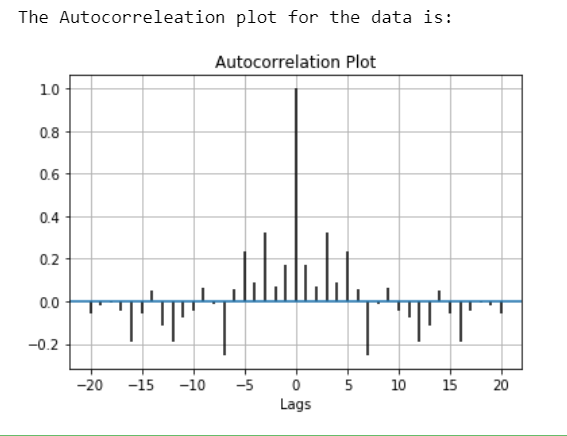# Autocorrelation plot using Matplotlib

Autocorrelation plots are a commonly used tool for checking randomness in a data set. This randomness is ascertained by computing autocorrelations for data values at varying time lags.

Characteristics Of Autocorrelation Plot :

• It measures a set of current values against a set of past values and finds whether they correlate.
• It is the correlation of one-time series data to another time series data which has a time lag.
• It varies from +1 to -1.
• An autocorrelation of +1 indicates that if time series one increases in value the time series 2 also increases in proportion to the change in time series 1.
• An autocorrelation of -1 indicates that if time series one increases in value the time series 2 decreases in proportion to the change in time series 1.

Application of Autocorrelation:

• Pattern recognition.
• Signal detection.
• Signal processing.
• Estimating pitch.
• Technical analysis of stocks.

## Plotting the Autocorrelation Plot

To plot the Autocorrelation Plot we can use matplotlib and plot it easily by using matplotlib.pyplot.acorr() function.

Syntax: matplotlib.pyplot.acorr(x, *, data=None, **kwargs)
Parameters:

• ‘x’ : This parameter is a sequence of scalar.
• ‘detrend’ : This parameter is an optional parameter. Its default value is mlab.detrend_none.
• ‘normed’ : This parameter is also an optional parameter and contains the bool value. Its default value is True.
• ‘usevlines’ : This parameter is also an optional parameter and contains the bool value. Its default value is True.
• ‘maxlags’ : This parameter is also an optional parameter and contains the integer value. Its default value is 10.
• ‘linestyle’ : This parameter is also an optional parameter and used for plotting the data points, only when usevlines is False.
• ‘marker’ : This parameter is also an optional parameter and contains the string. Its default value is ‘o’.

Returns: (lags, c, line, b)
Where:

• lags are a length 2`maxlags+1 lag vector.
• c is the 2`maxlags+1 auto correlation vector.
• line is a Line2D instance returned by plot.
• b is the x-axis.

Example 1:

## Python3

 `# Importing the libraries.` `import` `matplotlib.pyplot as plt ` `import` `numpy as np ` `  `  `# Data for which we plot Autocorrelation.` `data ``=` `np.array([``12.0``, ``24.0``, ``7.``, ``20.0``,` `                 ``7.0``, ``22.0``, ``18.0``,``22.0``,` `                 ``6.0``, ``7.0``, ``20.0``, ``13.0``,` `                 ``8.0``, ``5.0``, ``8``])` `  `  `# Adding plot title.` `plt.title(``"Autocorrelation Plot"``) `   `# Providing x-axis name.` `plt.xlabel(``"Lags"``) `   `# Plotting the Autocorrelation plot.` `plt.acorr(data, maxlags ``=` `10``) `   `# Displaying the plot.` `print``(``"The Autocorrelation plot for the data is:"``)` `plt.grid(``True``)`   `plt.show() `

Output:Example 2:

## Python3

 `# Importing the libraries.` `import` `matplotlib.pyplot as plt ` `import` `numpy as np ` `  `  `# Setting up the rondom seed for ` `# fixing the random state.` `np.random.seed(``42``) ` `  `  `# Creating some random data.` `data ``=` `np.random.randn(``25``) ` `  `  `# Adding plot title.` `plt.title(``"Autocorrelation Plot"``)`   `# Providing x-axis name.` `plt.xlabel(``"Lags"``)`   `# Plotting the Autocorrelation plot.` `plt.acorr(data, maxlags ``=` `20``) `   `# Displaying the plot.` `print``(``"The Autocorrelation plot for the data is:"``)` `plt.grid(``True``)`   `plt.show() `

Output:Whether you're preparing for your first job interview or aiming to upskill in this ever-evolving tech landscape, GeeksforGeeks Courses are your key to success. We provide top-quality content at affordable prices, all geared towards accelerating your growth in a time-bound manner. Join the millions we've already empowered, and we're here to do the same for you. Don't miss out - check it out now!